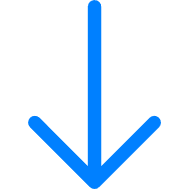QI will reread the problem if I don't understand it.

I will find all important
information in the problem and
click and highlight it.

Click and highlight all important information.

I will represent the problem.

 Touch or drag relevant numbers from the word problem.Represent the problem using the area model.Numerator Denominator Numerator Denominator

I will write the equation and answer it.

Write the equation and answer it.

 = = multiplier multiplicand Product
 QTouch or drag relevant numbers from the word problem.Represent the problem using the area model.Numerator Denominator Numerator Denominator
 = = multiplier multiplicand Product
X12345678910
112345678910
22468101214161820
336912151821242730
4481216202428323640
55101520253035404550
66121824303642485460
77142128354249566370
88162432404856647280
99182736455463728190
10102030405060708090100